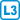## Voting preferences

### Task number: 2662

In a hypothetic town are three political parties: A, B and C. It is known that 75 % of voters of the party A will vote for them again, 5 % will vote for B and 20 % C. Similarly from those who voted for B will 60 % vote again for B, 20 % for A and 20 % for C. 80 % of voters of C will vote for them again, the remanining ballots will be distributed between 10 % for A and 10 % for B.

Determine the limit distribution of mandates in the municipal government (say with 100 representants)?

• #### Resolution

The change of ballots; distribution can be modelled by a linear map $$f:\mathbb R^3\to\mathbb R^3$$, given by the matrix $$\mathbf F$$. This matrix shall satisfy $$\mathbf x_{t+1}=f(\mathbf x_t)=\mathbf F\mathbf x_t$$, where $$x_t$$ menad the distribution of the ballots after the $$t$$-th voting round. $$\mathbf F=\begin{pmatrix} 0{,}75 & 0{,}20 & 0{,}10 \\ 0{,}05 & 0{,}60 & 0{,}10 \\ 0{,}20 & 0{,}20 & 0{,}80 \\ \end{pmatrix}$$

The matrix $$\mathbf F$$ is called stochastic, since it has all column sums 1. Observe that the associated linear map will preserve the sum of all components of any vector. In addition, the image of a vector with nonnegative components will also be nonnegative.

For the solution it suffices to verify that 1 is an eigenvalue of the matrix and find the corresponding eigenvector. In other words we shall solve the system $$\mathbf F\mathbf x=\mathbf x$$.

The fractions can be eliminated if we multiply the matrix by 20. (then the eigenvalues 20 times increase, but eigenvectors are preserved).

$$\mathbf F'=\begin{pmatrix} 15 & 4 & 2 \\ 1 & 12 & 2 \\ 4 & 4 & 16 \\ \end{pmatrix}$$

We may calculate all eigenvalues of $$\mathbf F'$$ and $$\mathbf F$$. These are $$\lambda_1'=20$$, $$\lambda_1=1$$, $$\lambda_2'=12$$, $$\lambda_2=12/20$$, and $$\lambda_3'=11$$, $$\lambda_3=11/20$$.

We look for an eigenvector associated with $$\lambda_1=1$$, with component sum 1. This is the limit distribution: $$\mathbf x = (1/3, 1/6, 1/2)^T \doteq (33\%, 17\%, 50\%)^T$$

What are the remaining eigenvectors? They must have zero componet sum as otherwise they could not be scaled by the eigenvalue and maintain this sum. Hence at least one of their component is negative.

The solutions of the associated systems are: $$\mathbf x=c\cdot(-2, 1, 1)^T,\mathbf x=c\cdot(-1, 1, 0)^T$$.

Why any initial distribution converges to the limit one? Consider the set of all probabilistic vectors, i.e. vectors from the convex hull of the canonic basis. These vectors have component sum 1 and have only nonnegative components. It is possible to show that the mapping $$f$$ is on this set a contraction, i.e. it decreases the norm of a difference of any two vectors. When $$f$$ is applied iteratively, any difference between images of two vectors can be bounded by a geometric sequence. This yilds existence and uniqueness of the limit. The limit indeed exists always when a contraction is applied on a compact set.